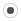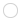## QR Decomposition and Sign of Determinantallanmulin
Registered MemberPosts
14
Karma
0

### QR Decomposition and Sign of Determinant

Wed May 30, 2012 7:02 pm
Hello, it seems that all QR decomposition in Eigen only provides the absolute value of the determinant of the decomposed matrix (the log of this value can also be queried). I understand that this simplifies considerably the calculation, since only the product of the diagonal entries of R is performed, neglecting the determinant of Q which is either +1 or -1.

However, I am facing a problem that requires the sign of the determinant (its absolute value is of no importance). This necessity can be found in continuation methods for non-linear problems.

So, how could I find efficiently (i.e. linear complexity O(n) if possible) the sign of the determinant of A, where AP=QR and P a permutation matrix?allanmulin
Registered MemberPosts
14
Karma
0

### Re: QR Decomposition and Sign of DeterminantWed May 30, 2012 8:59 pm
Okay, I will answer my own question in case someone needs this in the future. This is basically the approach discussed in the link below translated to Eigen.

http://icl.cs.utk.edu/lapack-forum/view ... f=2&t=1741

The determinant of Q is given by: det(Q) = (-1)^k, where k is the number of Householder reflections used in the QR decomposition. Assume:

HouseholderQR<MatrixXd> QR(A);

The number k is then the number of non-zero Householder coefficients in the vector QR.hCoeffs().ggael
ModeratorPosts
3447
Karma
19
OS### Re: QR Decomposition and Sign of Determinant

Thu May 31, 2012 10:21 pm
Thanks for sharing your finding. Patch welcome for adding true determinant() functions to the QR classes.

## Who is online

Registered users: Baidu [Spider], Bing [Bot], claydoh, farid, Google [Bot], jacksong, joebuckley, Moldmaker, Section_8, Sogou [Bot], zfazylz